# 3. Dry soil is mixed with water and compacted to produce a cylindrical sample (diameter= 38 mm, length = 76 mm) with a water content of 16.0 %. Air occupies 6 % of thetotal volume of the compacted sample. The specific gravity of soil solids is 2.71.Complete the phase diagram.

Question
11 views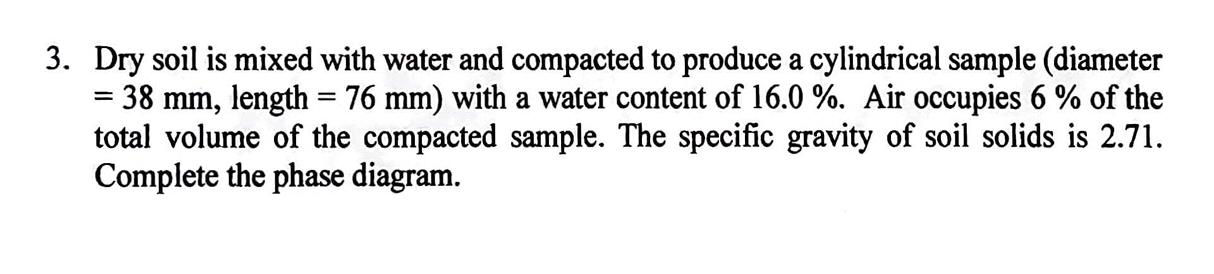help_outlineImage Transcriptionclose3. Dry soil is mixed with water and compacted to produce a cylindrical sample (diameter = 38 mm, length = 76 mm) with a water content of 16.0 %. Air occupies 6 % of the total volume of the compacted sample. The specific gravity of soil solids is 2.71. Complete the phase diagram. fullscreen
check_circle

Step 1

Given data:

Diameter of the cylindrical sample = 38 mm

Length of the cylindrical sample = 76 mm

Water content = 16%

Air content = 6%

Specific gravity of soil (G) = 2.71

Step 2

Calculate the Total volume (V):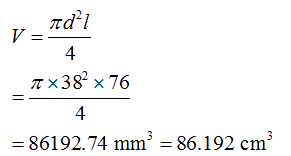Step 3

Calculate mass of solid (Ms):

Water content = 16%

Let M is the total mass of the soil

M = Ms + Mw

Mass of air is negligible, so it is neglected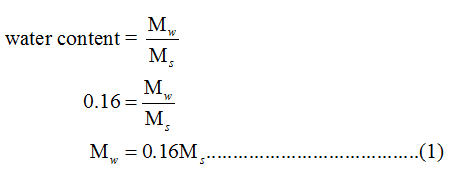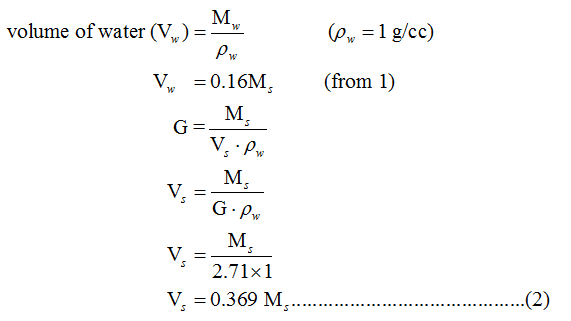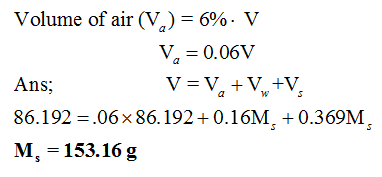...

### Want to see the full answer?

See Solution

#### Want to see this answer and more?

Solutions are written by subject experts who are available 24/7. Questions are typically answered within 1 hour.*

See Solution
*Response times may vary by subject and question.
Tagged in